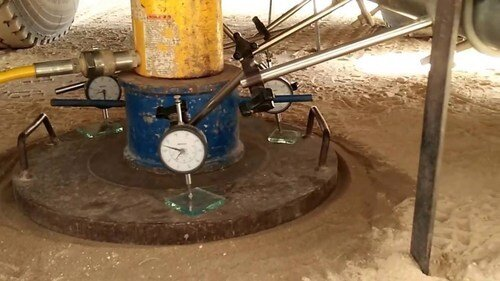# Modulus of Subgrade Reaction of SoilsThe modulus of subgrade reaction ks (also called the coefficient of subgrade reaction of soil) is the ratio of the pressure against a flat surface on soil and the settlement at that point. Mathematically, this is expressed as;

ks = q/δ ——- (1)

Where;
ks = Coefficient of subgrade reaction expressed in force/length2/length
q = pressure on the surface at the given point
δ = settlement at the same point

Against many popular opinions, the modulus of subgrade reaction is not an exclusive property of soil but depends mainly on the loaded area (size of the footing or mat). Other factors that affect the modulus of subgrade reaction are the shape of footing, depth of foundation, type of soil, and type of foundation. Different approaches have been suggested for the evaluation of the modulus of subgrade reaction, but the most practical approach is to carry out an in-situ plate load test on the soil.

Plate bearing test is an in-situ load-bearing test that is used to evaluate the ultimate bearing capacity and likely settlement of soil under a given load. The test can be carried out in accordance with BS 1377 Part 9: 1990. It basically consists of loading a steel plate of known diameter and recording the settlements corresponding to each load increment.

The test load is gradually increased till the plate starts to settle at a rapid rate. The total value of the load on the plate divided by the area of the steel plate gives the value of the ultimate bearing capacity of the soil. A factor of safety is applied to give the safe bearing capacity of the soil.

By implication, the coefficient of subgrade reaction is the unit pressure required to produce a unit settlement in soil. In saturated clay soils, the settlement under load will take time due to consolidation, so the coefficient of subgrade reaction should be determined on the basis of the final settlement.

On granular soil deposits, the settlement should take place immediately after the application of load. Therefore, the modulus of subgrade reaction is premised on two simplified assumptions which are;

• The value of ks is independent on the magnitude of the pressure
• The value of ks has the same value for every point on the surface of the footing

These two assumptions are however not strictly correct.

Terzaghi in 1955 presented empirical relationships for determining the coefficient of subgrade reactions (ksf) for full-scale foundations, based on results from plate load tests. This is based on a rigid 1 ft x 1 ft (0.305 m x 0.305m) slab placed on a soil medium.

(1) For a square footing on cohesionless soil (B x B);

ksf = ks[(B + 0.305)/2B]2 ——- (2)

(2) For a rectangular footing on cohesionless soil (B x L)

ksf,r = [ksf(1 + 0.5B/L)]/1.5 ——- (3)

(3) For a long strip footing of width B, the coefficient of subgrade reaction is approximately 0.67ksf

(4) For clay soils, it has been observed that the value of ks varies with the length of the footing. Therefore, for clays;

ksc = ks[(L + 0.152)/1.5L)] ——- (4)

Where;

ks = plate-load test value of modulus of subgrade reaction kN/m2/m, using square plate (1 × 1) ft or circular plate with diameter = 0.305 m
ksc = corrected plate-load test value of modulus of subgrade reaction kN/m2/m, using square plate (1 × 1) ft or circular plate with diameter = 0.305 m for clays
ksf = desired value of modulus of subgrade reaction for full-sized square footings B × B, kN/m2/m
ksf,r = desired value of modulus of subgrade reaction for rectangular full-sized footings B × L, kN/m2/m
B = footing width, or least dimension of rectangular or strip.
L = Length of footing

Some formulas suggested by different authors for evaluation of the modulus of subgrade reaction are given in the Table below;

ks = the coefficient of subgrade reaction. B’ = side dimension of square base used in the plate load test. B = width of footing. ks = the value of subgrade reaction for 0.3 × 0.3 (1 ft wide) bearing plate. ksf = value of modulus of subgrade reaction for the full-size foundation. Es = modulus of elasticity. μ = Poisson’s ratio. EI = flexural rigidity of footing, m = takes 1, 2 and 4 for edges, sides and center of footing, respectively. Is and If = influence factors depend on the shape and depth of footing.

One of the most popular relationships between allowable bearing capacity and modulus of subgrade reaction is given in equation (5) according to Bowles (1996);

ks = 40.(FS).(qa)  ——- (5)

Where qa is the allowable bearing capacity of the soil, and FS is the factor of safety that was used in converting the ultimate pressure (qult) to allowable pressure (qa). It is important to note that in equation (5), the author assumed a 25 mm settlement value for the soil.

Ping-Sien Lin, Li-Wen Yang, and C. Hsein Juang (1998) made a series of plate-load tests to investigate the load settlement characteristics of a gravelly cobble deposit and estimated the value of modulus of subgrade reaction ks as follows:

ks = qaa ——- (6)

Where;
ks = Coefficient of subgrade reaction
qa = Allowable bearing capacity = (ultimate bearing capacity/factor of safety)
δa = Allowable settlement

## Typical values of modulus of subgrade reaction

The typical values of modulus of subgrade reaction of different types of soils are given below;

#### 1 COMMENT

1.Sentayehu Dassew

It is very interesting and currently I found it very important for my project work in Geotechnical Engineering lesson.